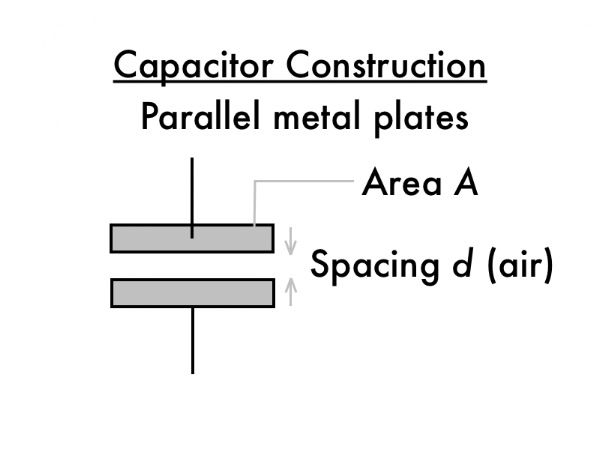## 20160311

Physics 205B, spring semester 2016
Cuesta College, San Luis Obispo, CA

Students have a bi-weekly online reading assignment (hosted by SurveyMonkey.com), where they answer questions based on reading their textbook, material covered in previous lectures, opinion questions, and/or asking (anonymous) questions or making (anonymous) comments. Full credit is given for completing the online reading assignment before next week's lecture, regardless if whether their answers are correct/incorrect. Selected results/questions/comments are addressed by the instructor at the start of the following lecture.

The following questions were asked on reading textbook chapters and previewing presentations on capacitors.Selected/edited responses are given below.

Describe what you understand from the assigned textbook reading or presentation preview. Your description (2-3 sentences) should specifically demonstrate your level of understanding.
"That electrons are the only ones that are moving in conductors. I assumed protons moved around as well, but I came to the conclusion that its just electrons."

"The basic model of a capacitor is very similar, with two parallel metal plates of equal area A, held a fixed distance d apart. To keep things simple we assume that the space between the plates is air."

"Capacitors hold charge through an electrical potential difference between two metal sheets. Changing the voltage input to the capacitor can change the storage on a capacitor, though each capacitor has a specific capacity based on the area of the metal plates and the distance between sheets."

"Capacitors can store EPE and voltage. Capacitors are made of two plates with fixed distance, where only electrons can move. Capacitors can release all of its charges very quickly as opposed to batteries."

"Capacitors store electric charge and thus energy. When a capacitor charges work is done on it by moving the electrons from one plate (+) to the other (-)."

Describe what you found confusing from the assigned textbook reading or presentation preview. Your description (2-3 sentences) should specifically identify the concept(s) that you do not understand.
"How to calculate the capacitance. I'm not sure if I quite understand the logic of using the equation."

"I would like to go through everything in general, I'm having a hard time grasping this section. I mainly want to go over what is happening within capacitors."

"How batteries and capacitors interact with each other from a physical standpoint."

"I understand that the batteries apply a potential to the capacitor allowing it to charge, but I guess I'm confused with the electron flow and how the battery facilitates that."

"All makes sense."

"Nope."

Describe two quantities that a capacitor is designed to store/hold.
"Voltage and electrical potential."

"The two quantities are positive charges and negative electric charges."

"It stores electric charges, and it stores electric potential energy in the form of those electric charges."

State the unit of capacitance, and give its definition in terms of other SI units.
"Farads, which equals coulombs per volt."

"Farads, which are coulombs-squared per joule."For a parallel-plate capacitor, ___________ the plate area and __________ the plate separation would increase its capacitance.
 decreasing; decreasing.  decreasing; increasing. **  increasing; decreasing. ************************  increasing; increasing. *  (Unsure/guessing/lost/help!) ****** 

For a parallel-plate capacitor, increasing the voltage (electric potential) difference applied to the capacitor would __________ the amount of charge stored in it.
 decrease. *****  increase. ***********************  have no effect on. ***  (Unsure/guessing/lost/help!) ** 

Explain why increasing or decreasing the voltage (electric potential difference) of a capacitor cannot change the numerical value of its capacitance.
"Capacitance is based on the built parameters of the capacitor. Without changing its construction, C cannot be changed."

"Because the voltage isn't even included in the capacitor equation."

"Because the charge stored would increase/decrease in direct proportion to the increase/decrease in voltage."

"The plate area and plate separation are fixed so the capacitance is unchanged."

"Not sure how to explain this."

":O"

Ask the instructor an anonymous question, or make a comment. Selected questions/comments may be discussed in class.
"I'm a little confused as to how you can change the amount of charge stored in a given capacitor, yet its capacitance remains fixed." (The "capacitance" of a capacitor is a proportionality "rating" of how much charge it can hold for a given amount of voltage applied. So if a certain capacitor is attached to a 1.5 V battery, it can hold 5.0 µC of charge. If instead a 9.0 V battery is attached to this capacitor (six times more voltage), then it will hold six times more charge, or 30 µC. You can't change this ratio of voltage to charge, unless you use a different capacitor, or somehow change how the capacitor was constructed.)

"Nothing I'm getting very frustrated with the topics presented. I can't read the book and just understand this stuff. I don't think enough explanation is given in class." (You're not alone. I don't think anyone can just read the textbook at first and understand this stuff. But we'll start with practicing the math in class (even if the concepts are a little shaky right now), and then hopefully with more context from the calculations the concepts will start to make more sense. This electricity stuff is very conceptually opaque, so go through this stuff at a slightly slower pace for this part of the semester. Just keep asking questions in class, lab, office hours, and/or e-mail.)